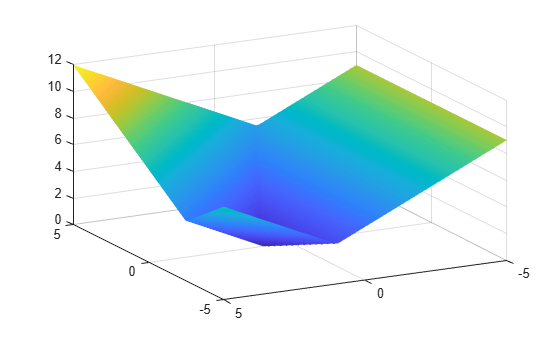# Compare `fminimax` and `fminunc`

A minimax problem minimizes the maximum of a set of objective functions. Why not minimize this maximum function, which is a scalar function? The answer is that the maximum is not smooth, and Optimization Toolbox™ solvers such as `fminunc` require smoothness.

For example, define `fun(x)` as three linear objective functions in two variables, and `fun2` as the maximum of these three objectives.

```a = [1;1]; b = [-1;1]; c = [0;-1]; a0 = 2; b0 = -3; c0 = 4; fun = @(x)[x*a+a0,x*b+b0,x*c+c0]; fun2 = @(x)max(fun(x),[],2);```

Plot the maximum of the three objectives.

```[X,Y] = meshgrid(linspace(-5,5)); Z = fun2([X(:),Y(:)]); Z = reshape(Z,size(X)); surf(X,Y,Z,'LineStyle','none') view(-118,28)````fminimax` finds the minimax point easily.

```x0 = [0,0]; [xm,fvalm,maxfval] = fminimax(fun,x0)```
```Local minimum possible. Constraints satisfied. fminimax stopped because the size of the current search direction is less than twice the value of the step size tolerance and constraints are satisfied to within the value of the constraint tolerance. ```
```xm = 1×2 -2.5000 2.2500 ```
```fvalm = 1×3 1.7500 1.7500 1.7500 ```
```maxfval = 1.7500 ```

However, `fminunc` stops at a point that is far from the minimax point.

`[xu,fvalu] = fminunc(fun2,x0)`
```Local minimum possible. fminunc stopped because it cannot decrease the objective function along the current search direction. ```
```xu = 1×2 0 1.0000 ```
```fvalu = 3.0000 ```

`fminimax` finds a better (smaller) solution.

`fprintf("fminimax finds a point with objective %g,\nwhile fminunc finds a point with objective %g.",maxfval,fvalu)`
```fminimax finds a point with objective 1.75, while fminunc finds a point with objective 3. ```